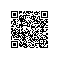# cheerp 减小调用转换类型的开销的方式

Cheerp提供了一些API来将C ++数组转换为底层的Typed Array对象。这对于将C ++数据直接传递给WebGL等浏览器API特别有用。

## 转换API

TypedArrayForPointerType
template < typename Tstruct TypedArrayForPointerType ;

TypedArrayForPointerType < unsigned short > :: type

char - > Int8Array
unsigned char - > Uint8Array

unsigned short - > Uint16Array
int - > Int32Array
unsigned int - > Uint32Array
float - > Float32Array
double - > Float64Array
MakeTypedArray

template < typename P，typename T = typename TypedArrayForPointerType

:: type>
T MakeTypedArray（const P ptr，size_t size = 0）

template < typename T>
T MakeTypedArray（const void ptr，size_t size = 0）

MakeArrayBufferView
client :: ArrayBufferView MakeArrayBufferView（const void ptr，size_t size = 0）

## 使用例子

static ArrayBuffer myJsSayHello (uint8 buffer) {

    Uint8Array * t = (Uint8Array *)cheerp::MakeArrayBufferView(buffer);
auto array = cheerp::makeArrayRef(t);

for( int i = 0 ; i < array->get_length(); i++ ) {
array[i] = array[i] + 100 ;
*(buffer+i) = array[i];
}
//{age:20, c:{age:21},hellow:"cheerp"}
struct Data d ;
d.age = 20;
d.c   = {21};
memcpy(d.hellow ,"cheerp", 7);
myCppSayHello(d);///js调用wasm
return cheerp::MakeArrayBufferView(array);

}使用钉钉扫一扫加入圈子
+ 订阅